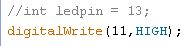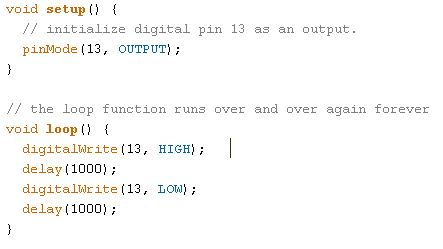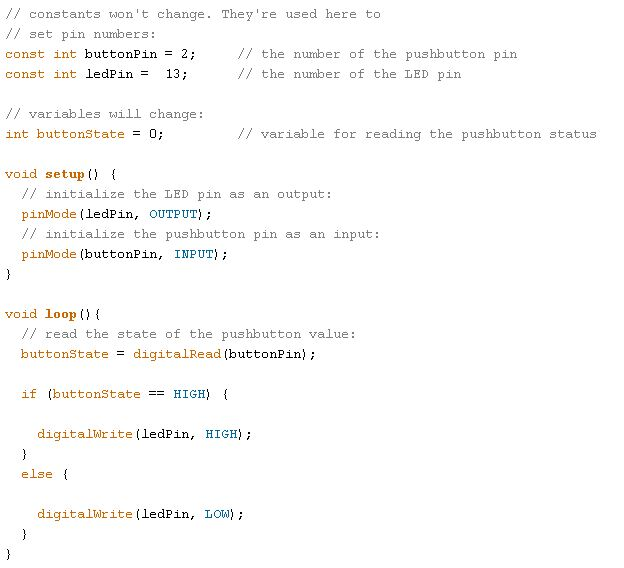Code test 1
This test allows you to autograde yourself about what you've learned with makit's activities and tutorials.
Name: *
How did you know us? *
Required
1. What do we need to program an Arduino board?
1 point
2. Which of the following are programming languages?
1 point
3. What are the parts of the basic structure of an Arduino program?
1 point
4. What is the main use of the setup function?
1 point
5. The different instructions we write on loop function…
1 point
6. Which of the following instructions would turn a led on? (led connected to pin 11)
1 point
7. If we want to pause the program for a second, we can use…
1 point
1 point
9. How do you declare a variable in Arduino?
1 point
10. Which ones of the following variable declarations are NOT correct?
1 point
11. What type of variable would you use to store your name?
1 point
12. What type of variable would you use to store a temperature value with decimals?
1 point
13. What type of variable would you use to store a button‘s state?
1 point
14. Select the function declaration that is correct
1 point
15. Considering a function called blink_function, how would you call it from loop function?
1 point
16. What’s the main difference between a conventional led and a RGB led?
1 point
17. What is an if statement?
1 point
18. What’s the difference between an analog and a digital input?
1 point
19. Which of the following could be uses for a potentiometer?
1 point
20. How many different colors can we achieve with a RGB led?
1 point
21. If we connect leds to each of the digital outputs of UNO board, how many leds would we be turning on with this for statement? For (int led = 0; led < 8; led++){ digitalWrite (led, HIGH);}
1 point
1 point
23. Comments are used by Arduino board...
1 point
24. What does portion 1 of code below do?
1 point
Portion of code 125. What does portion 2 of code below do?
1 point
Portion of code 226. What does portion 3 of code below do?
1 point
Portion of code 3